# What is Aperture Effect and How to Reduce it

## Aperture Effect

The spectrum of flat top sampled signal is expressed as,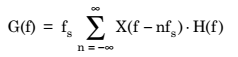……………. (1)

This equation shows that the signal g(t) is obtained by passing the signal s(t) through a filter having transfer function H(f).

The corresponding impulse response h(t) in time-domain has been shown in fig.1 (a).Fig.1: (a) One pulse of rectangular pulse train (b) spectrum of the pulse shown in figure (a)

Each sample of x(t) [i.e., s(t)] is convolved with this pulse.

Equation (1) represents that the spectrum of this rectangular pulse is multiplied with that of s(t).

Fig.1(b) shows the spectrum of one rectangular pulse of h(t).

We know that the spectrum of a rectangular pulse is expressed as,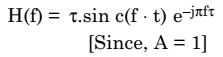………………. (2)

Hence, from fig.1(b), it may be observed that by using flat top samples an amplitude distortion is introduced in the reconstructed signal x(t) from g(t).

In fact, the high frequency roll-off of H(f) acts like a low-pass filter and thus attenuates the upper portion of message signal spectrum.

These high frequencies of x(t) are affected. This type of effect is known as aperture effect.

Now, as the duration ‘τ’ of the pulse increases, the aperture effect is more prominent. Hence, during reconstruction an equalizer is needed to compensate for this effect.

As shown in fig.2, the receiver contains a low-pass reconstruction filter with cutoff frequency slightly higher than the maximum frequency present in the message signal. The equalizer compensates for the aperture effect. It also compensates for the attenuation by a low-pass reconstruction filter.Fig.2 : Recovering x(t) at receiver

From equation (2), it may be noted that the sample function h(t) acts like a low-pass filter where Fourier transform is expressed as,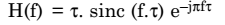This spectrum has been plotted in fig.1 (b).

Equalizer used in cascade with the reconstruction filter has the effect of decreasing the in band loss of the reconstruction filter as the frequency increases in such a way as to compensate for the aperture effect. Also, the transfer function of the equalizer is expressed as,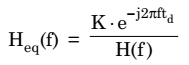Here ‘td’ is known as the delay introduced by low-pass filter which is equal to τ/2. Therefore,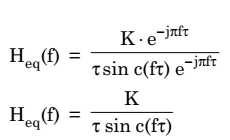which is the transfer function of an equalizer.